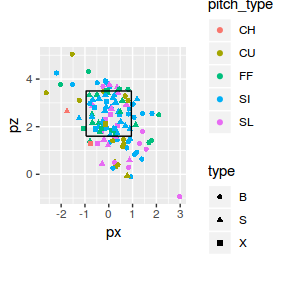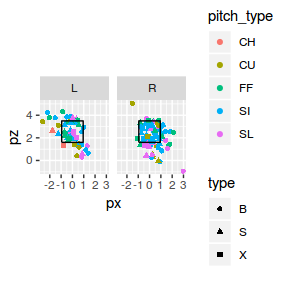# mlbgameday: Plotting Pitches

#### 2019-04-02

The package is primarily a data package, and has no native plotting tools. However, there are several plotting options available by leveraging one of the R language’s excellent plotting libraries.

## Example Data

For all of the following examples, we will use The pitch data for Jake Arrieta’s no-hitter, which occurred on April 21, 2016.

library(mlbgameday)
library(dplyr)
##
## Attaching package: 'dplyr'
## The following objects are masked from 'package:stats':
##
##     filter, lag
## The following objects are masked from 'package:base':
##
##     intersect, setdiff, setequal, union
# Grap some Gameday data. We're specifically looking for Jake Arrieta's no-hitter.
gamedat <- get_payload(start = "2016-04-21", end = "2016-04-21")
## Gathering Gameday data, please be patient...
# Subset that atbat table to only Arrieta's pitches and join it with the pitch table.
pitches <- inner_join(gamedat$pitch, gamedat$atbat, by = c("num", "url")) %>%
subset(pitcher_name == "Jake Arrieta")

## Ggplot2

The ggplot2 package can be used stand-alone, or in conjunction with Carson Silvert’s pitchRx package, which has additional visualization offerings that are based on ggplot2.

library(ggplot2)

# basic example
ggplot() +
geom_point(data=pitches, aes(x=px, y=pz, shape=type, col=pitch_type)) +
coord_equal() + geom_path(aes(x, y), data = mlbgameday::kzone)## Batting Stance

Using the same simple ggplot example, we can use facet_grid(. ~ stand) to segment the pitches thrown to right-handers from those thrown to left-handers.

# basic example with stand.
ggplot() +
geom_point(data=pitches, aes(x=px, y=pz, shape=type, col=pitch_type)) +
facet_grid(. ~ stand) + coord_equal() +
geom_path(aes(x, y), data =  mlbgameday::kzone)